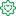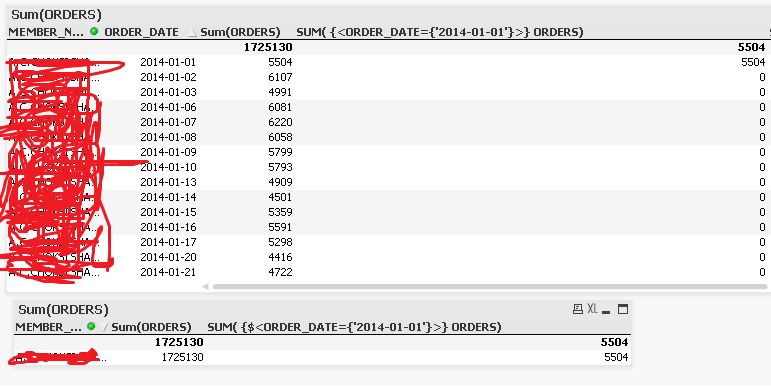Announcements
The way to achieve your own success is the willingness to help somebody else. Go for it!
cancel
Showing results for
Did you mean:Not applicable

## General Question on SET Analysis- Limitation

I have observe that when we use SET Analysis we mostly use the Column which is not part of the table Dimension ( table Column). For example if i have a Date Field on particular table and Date is part of table Dimension then if we want to use some SET Analysis based Expression and want Date Dimension ( Field) to be the condition.

If we do so then i found its not work as per the standard, but if we replace the Date Dimension to any other Dimension like Client Name, Address or any except the Field which is in SET Analysis its work as per the standard.

Can anyone have any idea why it is so ? or what is the best way to do so..

1 Solution

Accepted SolutionsFormer Employee

As I see it, QlikView is doing the right thing.

Both charts show that

Sum(ORDERS) = 1725130

Sum({<ORDER_DATE={'2014-01-01'}>} ORDERS) = 5504

When you add ORDER_DATE as dimension, the above numbers will be distributed over the dates, each dollar assigned to the proper date. So of course, you get 0 for all dates but one.

If you instead want every line to have 5504 on it, you need to use the total qualifier, e.g.

Sum({<ORDER_DATE={'2014-01-01'}>} total ORDERS)

or

Sum({<ORDER_DATE={'2014-01-01'}>} total <MEMBER_N...> ORDERS)

depending on which number you want (total over all MEMBER_Ns or total within each MEMBER_N)

HIC

The Aggregation Scope

When should the Aggr() function NOT be used?

4 RepliesFormer Employee

It does not matter whether the field in the Set modifier is used as dimension or not. The Set modifier works the same way in both cases.

If you have a case where you think that the Set Analysis isn't evaluated correctly, then please post an example that shows this.

HICNot applicable
Author

Dear Henric,

Below is my sample Report

when i create a tabular Report like Memberwise sum(Orders) and Sum(orders) of specific Date its work fine. ( See bottom one record table. its showing sum of orders and third column showing orders on particular date.

but when i just add order_date as dimension into the table its did not work as per need. means each datewise record i want 4th column should be the sum of the date which i have used in the filter.Former Employee

As I see it, QlikView is doing the right thing.

Both charts show that

Sum(ORDERS) = 1725130

Sum({<ORDER_DATE={'2014-01-01'}>} ORDERS) = 5504

When you add ORDER_DATE as dimension, the above numbers will be distributed over the dates, each dollar assigned to the proper date. So of course, you get 0 for all dates but one.

If you instead want every line to have 5504 on it, you need to use the total qualifier, e.g.

Sum({<ORDER_DATE={'2014-01-01'}>} total ORDERS)

or

Sum({<ORDER_DATE={'2014-01-01'}>} total <MEMBER_N...> ORDERS)

depending on which number you want (total over all MEMBER_Ns or total within each MEMBER_N)

HIC

The Aggregation Scope

When should the Aggr() function NOT be used?Not applicable
Author

Hi Henric,

Thanks you very much Man, i was struggling on this from 2-3 Days, i can't express my feeling you have solved my very big issue..

Thanks once again

Thanks & Regards,Community Browser